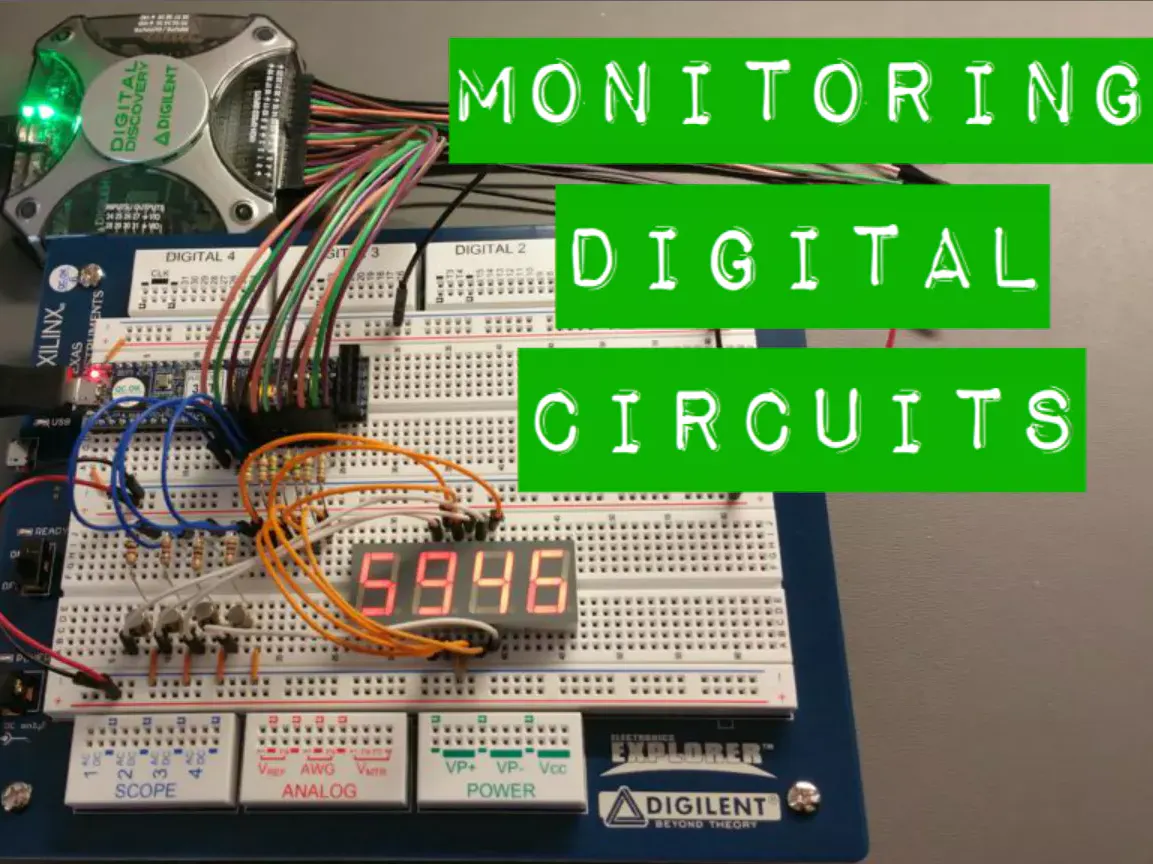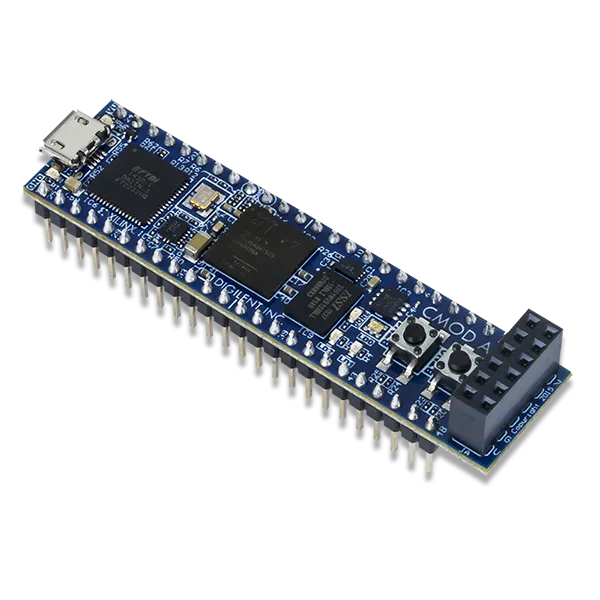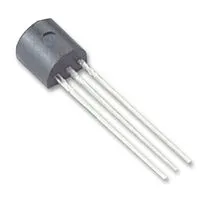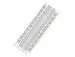# Monitoring Digital Circuits With the Digital Discovery

Monitoring digital circuits can be difficult at best. Sometimes it's nigh impossible. But the Digital Discovery makes it easier!

IntermediateFull instructions provided2 hours338## Things used in this project

### Hardware componentsDigilent Digital Discovery
×1Digilent Cmod A7 The A7 was chosen specifically because it has very few built-in peripheral devices on the board, i.e. no 7-segment LED display. As mentioned, any programmable chip and board will work for this.
×1General Purpose Transistor NPN I used 2N2222A, but this project won't be picky with a specific NPN version. These are used to control the cathode signals. The FPGA pins are not meant to drive high power loads, and while an LED might not seem like much, it's good practice to protect your chip FPGA by buffering the signal with a transistor. If you are using a common-anode display you will need to use PNP transistors on the anodes.
×4Solderless Breadboard Full Size Whatever works to make connections on the bread-board. Depending on your setup, you may need to use a couple of bread-boards.
×1
 7-segment LED display I used a common-cathode MSQ6941C, but a common-anode version will work with some design tweaks.
×1Resistor 1k ohm 5X 1kΩ resistors, one for each BJT base pin and one for the decimal point pin on the 7-segment display since it won't be used and can be driven low to keep it turned off.
×5
 Resistor 150 ohm 7X 150Ω resistors, one for each segment anode pin on the display.
×7

### Software apps and online servicesDigilent WaveForms 2015 This is a free software download and is required to interface with the Digital Discovery.

## Code

### Code snippet #1

Plain text
```module clkdiv(
input wire mclk,
input wire clr,
output wire clk183,
output wire clk6
);

reg [23:0] q;

always @(posedge mclk or posedge clr)
begin
if(clr == 1)
q <= 0;
else
q <= q + 1;
end

assign clk183 = q;  //183Hz
assign clk6 = q;   //6hz
endmodule
```

### Code snippet #2

Plain text
```module mod10kcount(
input wire clr,
input wire clk,
output reg [13:0] q
);

always @(posedge clk or posedge clr)
begin
if(clr == 1)
q <= 0;
else if(q == 9999)
q <= 0;
else
q <= q + 1;
end
endmodule
```

### Code snippet #3

Plain text
```module binbcd14(
input wire [13:0] b,
output reg [16:0] p
);

reg [32:0] z;
integer i;

always @(*)
begin
for(i = 0; i <= 32; i = i + 1)
z[i] = 0;
z[16:3] = b;

repeat(11)
begin
if(z[17:14] > 4)
z[17:14] = z[17:14] + 3;
if(z[21:18] > 4)
z[21:18] = z[21:18] + 3;
if(z[25:22] > 4)
z[25:22] = z[25:22] + 3;
if(z[29:26] > 4)
z[29:26] = z[29:26] + 3;
z[32:1] = z[31:0];
end
p = z[30:14];
end
endmodule
```

### Code snippet #4

Plain text
```module x7segbc(
input wire [15:0] x,
input wire cclk,
input wire clr,
output reg [6:0] a_to_g,
output reg [3:0] cath
);

reg [1:0] s;
reg [3:0] digit;
wire [3:0] cen;

assign cen = x | x | x | x;
assign cen = x | x | x | x | x | x | x | x;
assign cen = x | x | x | x | x | x | x | x | x | x | x | x;
assign cen = 1;

always @(*)
case(s)
0: digit = x[3:0];
1: digit = x[7:4];
2: digit = x[11:8];
3: digit = x[15:12];
default: digit = 4'b0000;
endcase

always @ (*)
case (digit) //gfedcba '1' = seg_on
0: a_to_g = 7'b0111111;
1: a_to_g = 7'b0000110;
2: a_to_g = 7'b1011011;
3: a_to_g = 7'b1001111;
4: a_to_g = 7'b1100110;
5: a_to_g = 7'b1101101;
6: a_to_g = 7'b1111101;
7: a_to_g = 7'b0000111;
8: a_to_g = 7'b1111111;
9: a_to_g = 7'b1101111;
'hA: a_to_g = 7'b1110111;
'hb: a_to_g = 7'b1111100;
'hC: a_to_g = 7'b1011000;
'hd: a_to_g = 7'b1011110;
'hE: a_to_g = 7'b1111001;
'hF: a_to_g = 7'b1110001;
endcase

always @(*)
begin
cath = 4'b0000;
if(cen[s] == 1)
an[s] = 1;
end

always @(posedge cclk or posedge clr)
begin
if(clr == 1)
s <= 0;
else
s <= s + 1;
end

endmodule
```

### Code snippet #5

Plain text
```module mod10kcount_top(
input wire sysclk,
input wire btn,
output wire [6:0] seg,
output wire [3:0] cath,
output wire led0_r,
output wire led0_g,
output wire led0_b
);

wire [16:0] p;
wire clr, clk6, clk183;
wire [13:0] b;

assign clr = btn;
assign led0_b = 1;
assign led0_g = 1;
assign led0_r = 1;

clkdiv U1 (
.mclk(sysclk),
.clr(clr),
.clk183(clk183),
.clk6(clk6)
);

mod10kcount U2 (
.clr(clr),
.clk(clk6),
.q(b)
);

binbcd14 U3 (
.b(b),
.p(p)
);

x7segbc U4 (
.x(p[15:0]),
.cclk(clk183),
.clr(clr),
.a_to_g(seg),
.cath(cath[3:0])
);

endmodule
```

### Code snippet #6

Plain text
```# Clock signal 12 MHz
set_property -dict { PACKAGE_PIN L17   IOSTANDARD LVCMOS33 } [get_ports { sysclk }]; #IO_L12P_T1_MRCC_14 Sch=gclk
create_clock -add -name sys_clk_pin -period 83.33 -waveform {0 41.66} [get_ports {sysclk}];

# LEDs
set_property -dict { PACKAGE_PIN B17   IOSTANDARD LVCMOS33 } [get_ports { led0_b }]; #IO_L14N_T2_SRCC_16 Sch=led0_b
set_property -dict { PACKAGE_PIN B16   IOSTANDARD LVCMOS33 } [get_ports { led0_g }]; #IO_L13N_T2_MRCC_16 Sch=led0_g
set_property -dict { PACKAGE_PIN C17   IOSTANDARD LVCMOS33 } [get_ports { led0_r }]; #IO_L14P_T2_SRCC_16 Sch=led0_r

# Buttons
set_property -dict { PACKAGE_PIN A18   IOSTANDARD LVCMOS33 } [get_ports { btn }]; #IO_L19N_T3_VREF_16 Sch=btn

# 7-seg
set_property -dict { PACKAGE_PIN U4    IOSTANDARD LVCMOS33 } [get_ports { cath }]; #IO_L11P_T1_SRCC_34 Sch=pio
set_property -dict { PACKAGE_PIN V5    IOSTANDARD LVCMOS33 } [get_ports { cath }]; #IO_L16N_T2_34 Sch=pio
set_property -dict { PACKAGE_PIN W4    IOSTANDARD LVCMOS33 } [get_ports { cath }]; #IO_L12N_T1_MRCC_34 Sch=pio
set_property -dict { PACKAGE_PIN U5    IOSTANDARD LVCMOS33 } [get_ports { cath }]; #IO_L16P_T2_34 Sch=pio

set_property -dict { PACKAGE_PIN U2    IOSTANDARD LVCMOS33 } [get_ports { seg }]; #IO_L9N_T1_DQS_34 Sch=pio
set_property -dict { PACKAGE_PIN W6    IOSTANDARD LVCMOS33 } [get_ports { seg }]; #IO_L13N_T2_MRCC_34 Sch=pio
set_property -dict { PACKAGE_PIN U3    IOSTANDARD LVCMOS33 } [get_ports { seg }]; #IO_L9P_T1_DQS_34 Sch=pio
set_property -dict { PACKAGE_PIN U7    IOSTANDARD LVCMOS33 } [get_ports { seg }]; #IO_L19P_T3_34 Sch=pio
set_property -dict { PACKAGE_PIN W7    IOSTANDARD LVCMOS33 } [get_ports { seg }]; #IO_L13P_T2_MRCC_34 Sch=pio
set_property -dict { PACKAGE_PIN U8    IOSTANDARD LVCMOS33 } [get_ports { seg }]; #IO_L14P_T2_SRCC_34 Sch=pio
set_property -dict { PACKAGE_PIN V8    IOSTANDARD LVCMOS33 } [get_ports { seg }]; #IO_L14N_T2_SRCC_34 Sch=pio
```

## Credits

### Martha Migliacio

5 projects • 10 followers

### Alex Wong

5 projects • 21 followers
I work in Digilent and like creating projects

### Brandon Marcum

7 projects • 1 follower